﻿ 铺砌(3.6.3.6)中直线上的D-点数 On the Number of D-Points on a Line in the Tiling (3.6.3.6)

Vol.06 No.07(2017), Article ID:22554,12 pages
10.12677/AAM.2017.67109

On the Number of D-Points on a Line in the Tiling (3.6.3.6)

Lin Peng, Liping Yuan

College of Mathematics and Information Science, Hebei Normal University, Shijiazhuang Hebei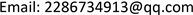Received: Oct. 8th, 2017; accepted: Oct. 24th, 2017; published: Oct. 31st, 2017ABSTRACT

A vertex of the Archimedean tiling (3.6.3.6) is called a D-point. In this paper, we first investigate the number of D-points lying on any given line in the plane, and prove that all the lines can be classified into three categories according to the numbers of D-points lying on them, namely, no D-point, one and only one D-point and an infinitely many D-points. We also give the whole characterizations of those three types of lines by some necessary and sufficient conditions. Furthermore, we consider the broadest paths that contain no D-points in their interiors in any given direction $\theta \in \left[0,\pi \right)$ .

Keywords:Archimedean Tiling, Lattice, Line, D-Point, Broadest Path1. 引言

2. 预备知识

${D}_{0}$ 为由向量 $u=\left(2,0\right)$ 与向量 $v=\left(1,\sqrt{3}\right)$ 生成的一般格，则有

${D}_{0}=\left\{pu+qv:p,q\in ℤ\right\}=\left\{\left(\frac{4p+2q}{2},\frac{2q\sqrt{3}}{2}\right):p,q\in ℤ\right\}.$

${D}_{0}$ 分别沿向量 $\left(\frac{1}{2},\frac{\sqrt{3}}{2}\right)$$\left(\frac{3}{2},\frac{\sqrt{3}}{2}\right)$ 平移得到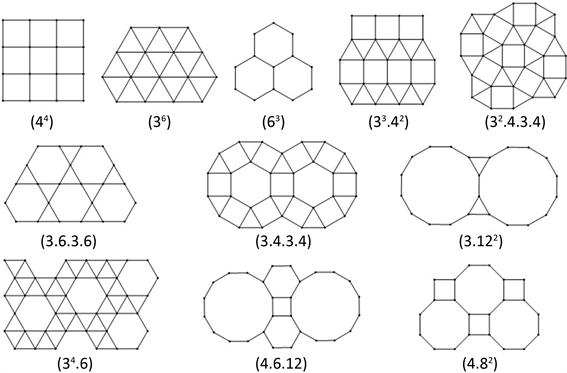Figure 1. Archimedean tilings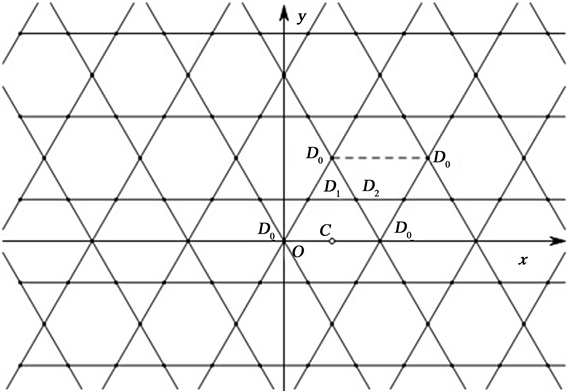Figure 2. Coordinate system

${D}_{1}=\left\{\left(\frac{1}{2},\frac{\sqrt{3}}{2}\right)+pu+qv:p,q\in ℤ\right\}=\left\{\left(\frac{4p+2q+1}{2},\frac{\left(2q+1\right)\sqrt{3}}{2}\right):p,q\in ℤ\right\},$

${D}_{2}=\left\{\left(\frac{3}{2},\frac{\sqrt{3}}{2}\right)+pu+qv:p,q\in ℤ\right\}=\left\{\left(\frac{4p+2q+3}{2},\frac{\left(2q+1\right)\sqrt{3}}{2}\right):p,q\in ℤ\right\}.$

$C=\left\{\left(1,0\right)+pu+qv:p,q\in ℤ\right\}=\left\{\left(\frac{4p+2q+2}{2},\frac{2q\sqrt{3}}{2}\right):p,q\in ℤ\right\}.$

${D}_{0}=\left\{\left(\frac{m}{2},\frac{n\sqrt{3}}{2}\right):m,n\in ℤ,\text{}m+n\equiv 0\text{}\left(\mathrm{mod}\text{4}\right),\text{}n\equiv 0\text{}\left(\mathrm{mod}\text{2}\right)\right\},$

${D}_{1}=\left\{\left(\frac{m}{2},\frac{n\sqrt{3}}{2}\right):m,n\in ℤ,\text{}m+n\equiv 2\text{}\left(\mathrm{mod}\text{4}\right),\text{}n\equiv 1\text{}\left(\mathrm{mod}\text{2}\right)\right\},$

${D}_{2}=\left\{\left(\frac{m}{2},\frac{n\sqrt{3}}{2}\right):m,n\in ℤ,\text{}m+n\equiv 0\text{}\left(\mathrm{mod}\text{4}\right),\text{}n\equiv 1\text{}\left(\mathrm{mod}\text{2}\right)\right\},$

$C=\left\{\left(\frac{m}{2},\frac{n\sqrt{3}}{2}\right):m,n\in ℤ,\text{}m+n\equiv 2\text{}\left(\mathrm{mod}\text{4}\right),\text{}n\equiv 0\text{}\left(\mathrm{mod}\text{2}\right)\right\}.$

$T=\left\{p\left(1,0\right)+q\left(\frac{1}{2},\frac{\sqrt{3}}{2}\right):p,q\in ℤ\right\}=\left\{\left(\frac{2p+q}{2},\frac{q\sqrt{3}}{2}\right):p,q\in ℤ\right\},$

$T=\left\{\left(\frac{m}{2},\frac{n\sqrt{3}}{2}\right):m,n\in ℤ,\text{}m+n\equiv 0\text{}\left(\mathrm{mod}\text{2}\right)\right\}.$

(a) 若点 $P\in {D}_{1}$ ，则 $\alpha P\in {D}_{1},\beta P\in {D}_{0}$

(b) 若点 $P\in {D}_{2}$ ，则 $\alpha P\in {D}_{2}\text{,}\beta P\in {D}_{0}$

(c) 若点 $P\in C$ ，则 $\alpha P\in C,\beta P\in {D}_{0}$

(a) 若 $Q\in {D}_{0}$ ，则 ${D}_{i}$ -点 $\stackrel{Q}{\to }$ ${D}_{i}$ -点 $\left(i=0,1,2\right)$ ，C-点 $\stackrel{Q}{\to }$ C-点。

(b) 若 $Q\in {D}_{1}$ ，则 ${D}_{0}$ -点 $\stackrel{Q}{\to }$ ${D}_{1}$ -点， ${D}_{1}$ -点 $\stackrel{Q}{\to }$ ${D}_{0}$ -点， ${D}_{2}$ -点 $\stackrel{Q}{\to }$ C-点，C-点 $\stackrel{Q}{\to }$${D}_{2}$ -点。

(c) 若 $Q\in {D}_{2}$ ，则 ${D}_{0}$ -点 $\stackrel{Q}{\to }$ ${D}_{2}$ -点， ${D}_{2}$ -点 $\stackrel{Q}{\to }$ ${D}_{0}$ -点， ${D}_{1}$ -点 $\stackrel{Q}{\to }$ C-点，C-点 $\stackrel{Q}{\to }$ ${D}_{1}$ -点。

(d) 若 $Q\in C$ ，则 ${D}_{0}$ -点 $\stackrel{Q}{\to }$ C-点，C-点 $\stackrel{Q}{\to }$ ${D}_{0}$ -点， ${D}_{1}$ -点 $\stackrel{Q}{\to }$ ${D}_{2}$ -点， ${D}_{2}$ -点 $\stackrel{Q}{\to }$ ${D}_{1}$ -点。

(e) 若点 $P\notin T$ ，则点 $P+Q\notin T$

(a) 若 $Q\in {D}_{0}$ ，则 $m+n\equiv 0\text{}\left(\mathrm{mod}\text{}4\right)$$n\equiv 0\text{}\left(\mathrm{mod}\text{}2\right)$ 。于是 ${n}_{i}+n\equiv {n}_{i}\text{}\left(\mathrm{mod}\text{}2\right)$${m}_{i}+m+{n}_{i}+n\equiv {m}_{i}+{n}_{i}\text{}\left(\mathrm{mod}\text{}4\right)$ ，故点 ${P}_{i}{}^{\prime }$ 与点 ${P}_{i}$ 属于同一类型。因此，平移OQ后， ${D}_{i}$ -点变为 ${D}_{i}$ -点，C-点变为C-点。

(b) 若 $Q\in {D}_{1}$ ，则 $m+n\equiv 2\text{}\left(\mathrm{mod}\text{}4\right)$$n\equiv 1\text{}\left(\mathrm{mod}\text{}2\right)$ 。由于 ${n}_{0}+n\equiv 1\text{}\left(\mathrm{mod}\text{}2\right)$${m}_{0}+m+{n}_{0}+n\equiv 2\text{}\left(\mathrm{mod}\text{}4\right)$ ，则点 ${{P}^{\prime }}_{0}\in {D}_{1}$ ；由于 ${n}_{1}+n\equiv 0\text{}\left(\mathrm{mod}\text{}2\right)$${m}_{1}+m+{n}_{1}+n\equiv 0\text{}\left(\mathrm{mod}\text{}4\right)$ ，则点 ${{P}^{\prime }}_{1}\in {D}_{0}$ ；由于 ${n}_{2}+n\equiv 0\text{}\left(\mathrm{mod}\text{}2\right)$${m}_{2}+m+{n}_{2}+n\equiv 2\text{}\left(\mathrm{mod}\text{}4\right)$ ，则点 ${{P}^{\prime }}_{2}\in C$ ；由于 ${n}_{3}+n\equiv 1\text{}\left(\mathrm{mod}\text{}2\right)$${m}_{3}+m+{n}_{3}+n\equiv 0\text{}\left(\mathrm{mod}\text{}4\right)$ ，则点 ${P}_{3}{}^{\prime }\in {D}_{2}$ 。故平移OQ后， ${D}_{0}$ -点变为 ${D}_{1}$ -点， ${D}_{1}$ -点变为 ${D}_{0}$ -点， ${D}_{2}$ -点变为C-点，C-点变为 ${D}_{2}$ -点。

(e) 记点 ${P}^{\prime }=P+Q$ ，则点 $P={P}^{\prime }+\left(-Q\right)$ 。根据引理2.3，由点 $Q\in T$ 可得点 $-Q\in T$ ，于是综合(a)~(d)可得，若点 ${P}^{\prime }\in T$ ，则 ${P}^{\prime }+\left(-Q\right)\in T$ ，即点 $P\in T$ ，与条件点 $P\notin T$ 矛盾，故 $P+Q\notin T$

3. 平面内任意直线上的D-点数及其分布

$l$ 为平面内任意一条直线，若直线l的斜率k不存在，可设其方程为 $x=a\text{}\left(a\in ℝ\right)$ ，则显然有下述定理：

(a) ${N}_{l}\left(D\right)=\infty$ 当且仅当 $a=\frac{m}{2}$ ，其中 $m\in ℤ$

(b) ${N}_{l}\left(D\right)=0$ 当且仅当 $a\ne \frac{m}{2}$ ，其中 $m\in ℤ$

(a) ${N}_{l}\left(D\right)=\infty$ 当且仅当 $k\in \left\{\lambda \sqrt{3}:\lambda \in ℚ\right\}$

(b) ${N}_{l}\left(D\right)=1$ 当且仅当 $k\notin \left\{\lambda \sqrt{3}:\lambda \in ℚ\right\}$

(a) 必要性：由于 ${N}_{l}\left(D\right)=\infty$ ，则直线l上必含不同于点P的D-点 $Q$ 。令点 ${Q}^{\prime }=Q-P$ ，显然有点 ${Q}^{\prime }\in {l}^{\prime }$ ，根据引理2.3可得点 $-P\in D$ ，从而由引理2.4可得点 ${Q}^{\prime }\in T$ 。因为 $Q\ne P$ ，所以 ${Q}^{\prime }\ne O$ 。于是由定理3.2可得 ${N}_{{l}^{\prime }}\left(D\right)=\infty$ ，进而根据定理3.4可得直线 ${l}^{\prime }$ 的斜率即直线l的斜率 $k\in \left\{\lambda \sqrt{3}:\lambda \in ℚ\right\}$

(b) 必要性：假设直线l的斜率 $k\in \left\{\lambda \sqrt{3}:\lambda \in ℚ\right\}$ ，由(a)可得此时 ${N}_{l}\left(D\right)=\infty$ ，与已知条件 ${N}_{l}\left(D\right)=1$ 矛盾，因此 $k\notin \left\{\lambda \sqrt{3}:\lambda \in ℚ\right\}$

(1) 直线l具有性质 ${D}_{b}$

(2) $k\in \left\{\lambda \sqrt{3}:\lambda \in ℚ\right\}$

(1) 直线l具有性质 ${D}_{b}$

(2) $k\notin \left\{\lambda \sqrt{3}:\lambda \in ℚ\right\}$

(a) 直线l上仅含无穷多个 ${D}_{0}$ -点当且仅当 $m+n\equiv 1\text{}\left(\mathrm{mod}\text{}2\right)$ ；特别地，此时 ${N}_{l}\left(C\right)=\infty$

(b) ${N}_{l}\left({D}_{0}\right)={N}_{l}\left({D}_{1}\right)=\infty$${N}_{l}\left({D}_{2}\right)=0$ 当且仅当 $m+n\equiv 2\text{}\left(\mathrm{mod}\text{}4\right)$ ；特别地，此时 ${N}_{l}\left(C\right)=0$

(c) ${N}_{l}\left({D}_{0}\right)={N}_{l}\left({D}_{2}\right)=\infty$${N}_{l}\left({D}_{1}\right)=0$ 当且仅当 $m+n\equiv 0\text{}\left(\mathrm{mod}\text{}4\right)$ ；特别地，此时 ${N}_{l}\left(C\right)=0$

(a) 必要性：令点 $P=\left(\frac{m}{2},\frac{n\sqrt{3}}{2}\right)$ ，则点P在直线l上。若 $m+n\equiv 0\text{}\left(\mathrm{mod}\text{}2\right)$ ，则 $n\equiv 1\text{}\left(\mathrm{mod}\text{}2\right)$ ，于是当 $m+n\equiv 0\text{}\left(\mathrm{mod}\text{}4\right)$ 时点 $P\in {D}_{2}$ ；当 $m+n\equiv 2\left(\mathrm{mod}\text{}4\right)$ 时点 $P\in {D}_{1}$ 。从而直线l上必含 ${D}_{1}$ -点或 ${D}_{2}$ -点，与条件直线l上仅含 ${D}_{0}$ -点矛盾，因此 $m+n\equiv 1\text{}\left(\mathrm{mod}\text{}2\right)$

(b) 必要性：由于 ${N}_{l}\left({D}_{0}\right)={N}_{l}\left({D}_{1}\right)=\infty$ ，即直线l上不仅含 ${D}_{0}$ -点，则由(a)可得 $m+n\equiv /1\text{}\left(\mathrm{mod}\text{2}\right)$ 。于是有 $m+n\equiv 0\text{}\left(\mathrm{mod}\text{}2\right)$ ，此时 $m\equiv n\equiv 1\text{}\left(\mathrm{mod}\text{}2\right)$ ，从而有 $m+n\equiv 0\text{}\left(\mathrm{mod}\text{}4\right)$$m+n\equiv 2\text{}\left(\mathrm{mod}\text{}4\right)$ 。易验证点 $P=\left(\frac{m}{2},\frac{n\sqrt{3}}{2}\right)$ 在直线l上，若 $m+n\equiv 0\text{}\left(\mathrm{mod}\text{}4\right)$ ，则点 $P\in {D}_{2}$ ，与已知条件 ${N}_{l}\left({D}_{2}\right)=0$ 矛盾，因此 $m+n\equiv 2\text{}\left(\mathrm{mod}\text{}4\right)$

(a) 直线l上仅含无穷多个 ${D}_{0}$ -点当且仅当 $m+n\equiv 1\text{}\left(\mathrm{mod}\text{}2\right)$

(b) ${N}_{l}\left({D}_{0}\right)={N}_{l}\left({D}_{1}\right)=\infty$${N}_{l}\left({D}_{2}\right)=0$ 当且仅当 $m+n\equiv 2\text{}\left(\mathrm{mod}\text{}4\right)$

(c) ${N}_{l}\left({D}_{0}\right)={N}_{l}\left({D}_{2}\right)=\infty$${N}_{l}\left({D}_{1}\right)=0$ 当且仅当 $m+n\equiv 0\text{}\left(\mathrm{mod}\text{}4\right)$

(a) 直线l上仅含无穷多个 ${D}_{1}$ -点当且仅当 $m+n\equiv 0\text{}\left(\mathrm{mod}\text{}4\right)$

(b) ${N}_{l}\left({D}_{1}\right)={N}_{l}\left({D}_{0}\right)=\infty$${N}_{l}\left({D}_{2}\right)=0$ 当且仅当 $m+n\equiv 2\text{}\left(\mathrm{mod}\text{}4\right)$

(c) ${N}_{l}\left({D}_{1}\right)={N}_{l}\left({D}_{2}\right)=\infty$${N}_{l}\left({D}_{0}\right)=0$ 当且仅当 $m+n\equiv 1\text{}\left(\mathrm{mod}\text{}2\right)$

(a) 由定理3.13可知 ${N}_{{l}^{\prime }}\left({D}_{0}\right)={N}_{{l}^{\prime }}\left({D}_{2}\right)=\infty$${N}_{{l}^{\prime }}\left({D}_{1}\right)=0$ 当且仅当 $m+n\equiv 0\text{}\left(\mathrm{mod}\text{}4\right)$ ，且此时 ${N}_{{l}^{\prime }}\left(C\right)=0$ ，即 ${l}^{\prime }$ 上除了 ${D}_{0}$ -点及 ${D}_{2}$ -点以外，其它点均为非T-点。由引理2.4可得，将直线 ${l}^{\prime }$ 平移至直线l后， ${l}^{\prime }$ 上的 ${D}_{0}$ -点与 ${D}_{2}$ -点分别变为l上的 ${D}_{1}$ -点与C-点，而 ${l}^{\prime }$ 上的其它点均变为l上的非T-点，亦为非D-点，于是直线l上仅含无穷多个 ${D}_{1}$ -点。因此，直线l上仅含无穷多个 ${D}_{1}$ -点当且仅当 $m+n\equiv 0\text{}\left(\mathrm{mod}\text{}4\right)$

(b) 由定理3.13可知 ${N}_{{l}^{\prime }}\left({D}_{0}\right)={N}_{{l}^{\prime }}\left({D}_{1}\right)=\infty$${N}_{{l}^{\prime }}\left({D}_{2}\right)=0$ 当且仅当 $m+n\equiv 2\text{}\left(\mathrm{mod}\text{}4\right)$ ，且此时 ${N}_{{l}^{\prime }}\left(C\right)=0$ ，即 ${l}^{\prime }$ 上除了 ${D}_{0}$ -点及 ${D}_{1}$ -点以外，其它点均为非T-点。由引理2.4可得，将 ${l}^{\prime }$ 平移至l后， ${l}^{\prime }$ 上的 ${D}_{0}$ -点与 ${D}_{1}$ -点分别变为l上的 ${D}_{1}$ -点与 ${D}_{0}$ -点，而 ${l}^{\prime }$ 上的其它点均变为l上的非T-点，亦为非D-点，于是 ${N}_{l}\left({D}_{1}\right)={N}_{l}\left({D}_{0}\right)=\infty$${N}_{l}\left({D}_{2}\right)=0$ 。因此 ${N}_{l}\left({D}_{1}\right)={N}_{l}\left({D}_{0}\right)=\infty$${N}_{l}\left({D}_{2}\right)=0$ 当且仅当 $m+n\equiv 2\text{}\left(\mathrm{mod}\text{}4\right)$

(c) 由定理3.13可知直线 ${l}^{\prime }$ 上仅含无穷多个 ${D}_{0}$ -点当且仅当 $m+n\equiv 1\text{}\left(\mathrm{mod}\text{}2\right)$ ，且此时 ${N}_{{l}^{\prime }}\left(C\right)=\infty$ ，即 ${l}^{\prime }$ 上除了 ${D}_{0}$ -点及C-点以外，其它点均为非T-点。由引理2.4可得，将 ${l}^{\prime }$ 平移至l后， ${l}^{\prime }$ 上的 ${D}_{0}$ -点与C-点分别变为l上的 ${D}_{1}$ -点与 ${D}_{2}$ -点，而 ${l}^{\prime }$ 上的其它点均变为l上的非T-点，亦为非D-点，于是 ${N}_{l}\left({D}_{1}\right)={N}_{l}\left({D}_{2}\right)=\infty$${N}_{l}\left({D}_{0}\right)=0$ 。因此 ${N}_{l}\left({D}_{1}\right)={N}_{l}\left({D}_{2}\right)=\infty$${N}_{l}\left({D}_{0}\right)=0$ 当且仅当 $m+n\equiv 1\text{}\left(\mathrm{mod}\text{}2\right)$

(a) 直线l上仅含无穷多个 ${D}_{2}$ -点当且仅当 $m+n\equiv 2\text{}\left(\mathrm{mod}\text{}4\right)$

(b) ${N}_{l}\left({D}_{2}\right)={N}_{l}\left({D}_{0}\right)=\infty$${N}_{l}\left({D}_{1}\right)=0$ 当且仅当 $m+n\equiv 0\text{}\left(\mathrm{mod}\text{}4\right)$

(c) ${N}_{l}\left({D}_{2}\right)={N}_{l}\left({D}_{1}\right)=\infty$${N}_{l}\left({D}_{0}\right)=0$ 当且仅当 $m+n\equiv 1\text{}\left(\mathrm{mod}\text{}2\right)$

4. $\theta \in \left[0,\pi \right)$ 方向上内部无D-点的最宽路径

$\theta \in \left(0,\frac{\pi }{2}\right)\cup \left(\frac{\pi }{2},\pi \right)$ 时，按 $\mathrm{tan}\theta$ 是否具有形式 $\lambda \sqrt{3}\left(\lambda \in ℚ\right)$ 可以分为两种情形讨论。首先考虑 $\mathrm{tan}\theta \ne \lambda \sqrt{3}\left(\lambda \in ℚ\right)$ 的情形，对于直线 $l:y=kx+b$ ，其中 $k\notin \left\{\lambda \sqrt{3}:\lambda \in ℚ\right\}$ ，其至多含一个D-点，下面考虑l附近的D-点分布情况。

$r<{p}_{1}\alpha -{q}_{1} (1)

$r-\epsilon <{p}_{2}\alpha -{q}_{2} (2)

$0<{m}_{0}\frac{\sqrt{3}}{k}-{n}_{0}-\frac{b}{2k}<\frac{{\epsilon }_{1}}{2k},$

$0<2{m}_{0}\sqrt{3}-\left(k\cdot 2{n}_{0}+b\right)<{\epsilon }_{1}.$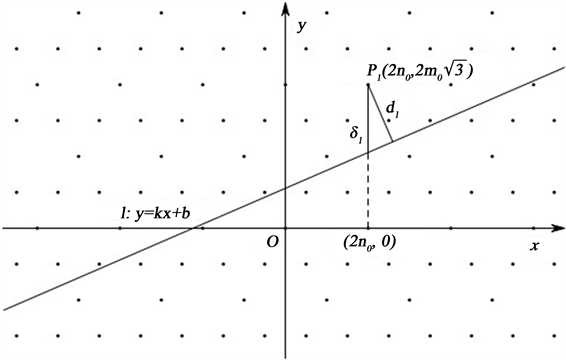Figure 3. Constructing D-point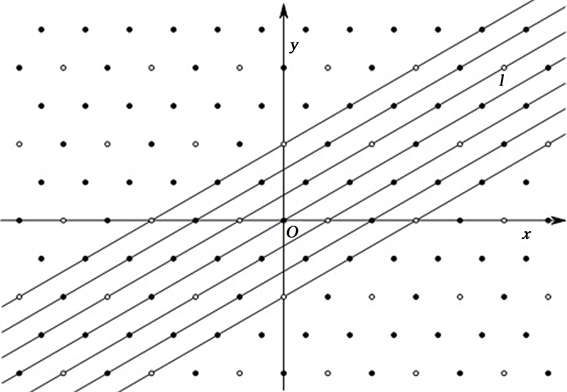Figure 4. The broadest paths containing no D-points in their interiors

${d}_{\theta }=\left\{\begin{array}{cc}\frac{\sqrt{3}}{\sqrt{3{n}^{2}+{m}^{2}}},& 若m,n\text{}均为奇数\\ \frac{\sqrt{3}}{2\sqrt{3{n}^{2}+{m}^{2}}},& 若m,n\text{}有一个为偶数\end{array}$ .

On the Number of D-Points on a Line in the Tiling (3.6.3.6)[J]. 应用数学进展, 2017, 06(07): 905-916. http://dx.doi.org/10.12677/AAM.2017.67109

1. 1. Grünbaum, B. and Shephard, G.C. (1987) Tilings and Patterns. W. H. Freeman and Company, New York.

2. 2. Olds, C.D., Lax, A. and Davidoff, G.P. (2001) The Geometry of Numbers. The Mathematical Association of America, Washington.

3. 3. Cao, P. and Yuan, L. (2014) The Number of Lattice Points and T-Points on a Line in . Southeast Asian Bulletin of Mathematics, 38, 21-25.

4. 4. 常之魁, 李英姿. 铺砌(33.42)中直线上的D-点数[J]. 数学杂志, 2013, 33(2): 359-362.

5. 5. 曹鹏浩. 关于H-点相关问题的研究[D]: [硕士学位论文]. 石家庄: 河北师范大学, 2010.